# 02.02.04: Pylon

## Example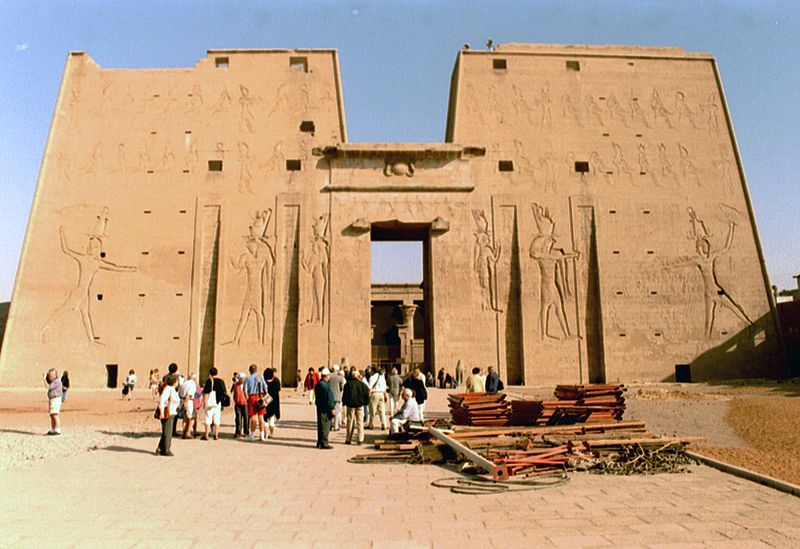Source: Wikipedia

## Where to Use

When desiring to evoke the Egyptian architectural element of a pylon as part of an overall structure.

## Solution

Consider using two pillars and an embedded lintel. The pillars may be slightly tapered.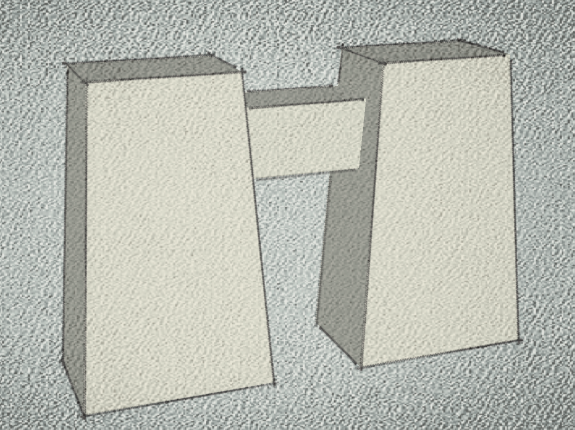What dimensions should be used to capture the look of ancient Egyptian pylons? Key elements are the slope of the sides of the pylon, along with their aspect ratios. This is illustrated nicely in the image below (source: Wikipedia):Measurements from several images show the slope is 6-7 degrees from vertical. Consider the following geometrical construction using Golden ratios: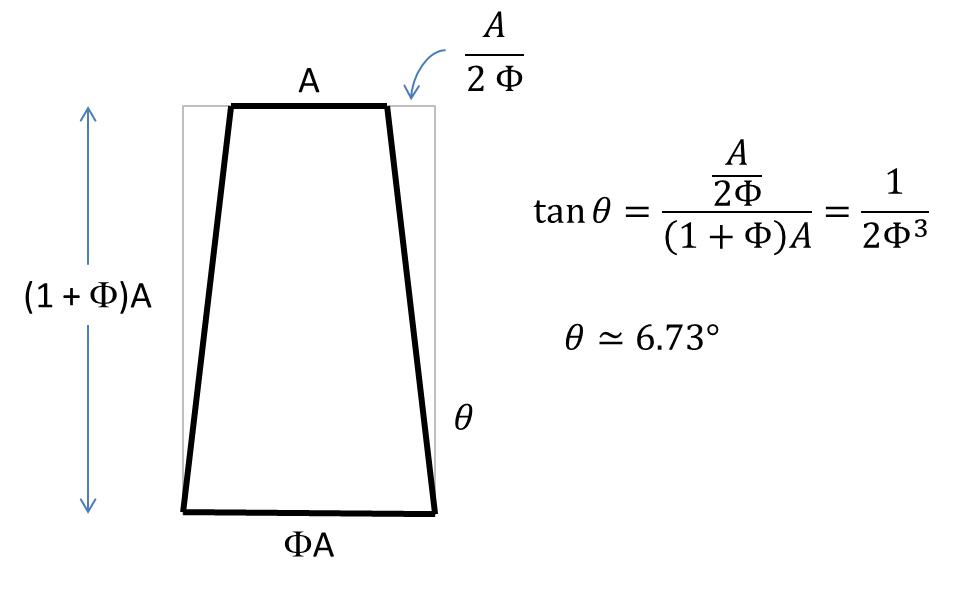Note that the top edge, base, and height are a Fibonacci sequence.

If the base of the pylon is a Golden rectangle, then the long side dimension needs to be the short side times a factor of Phi giving Phi*Phi*A = (1+Phi)*A. This is the height of the above construction. In order to have the same slope on the long face, the top edge must have a length of 2A (a rather nice result). The front face may also be constructed with the above geometry by scaling up all dimensions by Phi and then truncating the height equal to the base.

The overall dimensions for a complete pylon are shown below: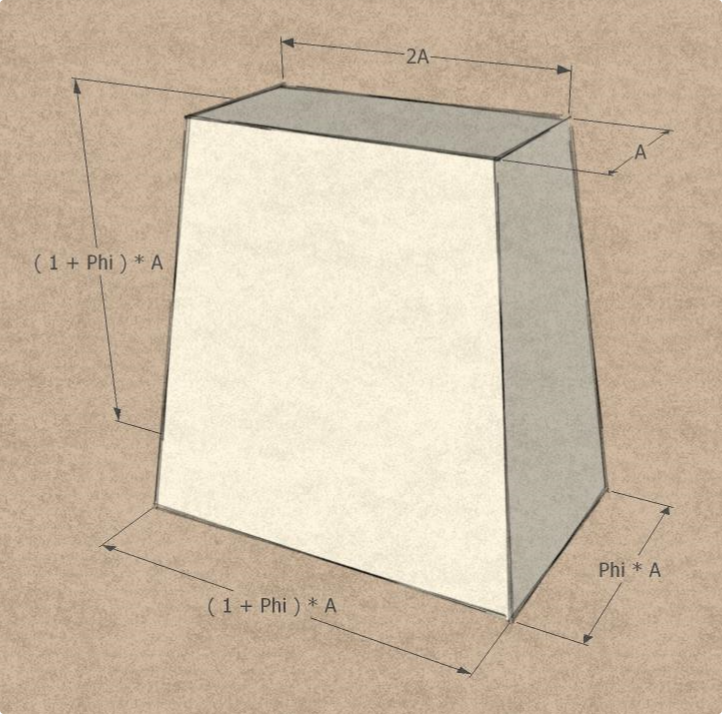Note that the height relates to the projection of a side (ie, the face is of course slightly longer due to its slope).

This construction uses Fibonacci sequences as described above; an excellent approximation can be obtained using a sequence of Fibonacci numbers F(n) multiplied by a base dimension D (creating preferred dimensions) to set the actual dimensions:

• Short top edge: D*F(n)
• Short base edge: D*F(n+1)
• Long base edge: D*F(n+2)

Noting that some ancient Egyptian pylons can be narrower on the short side. An alternate construction with a shorter side can be made that maintains the above slopes: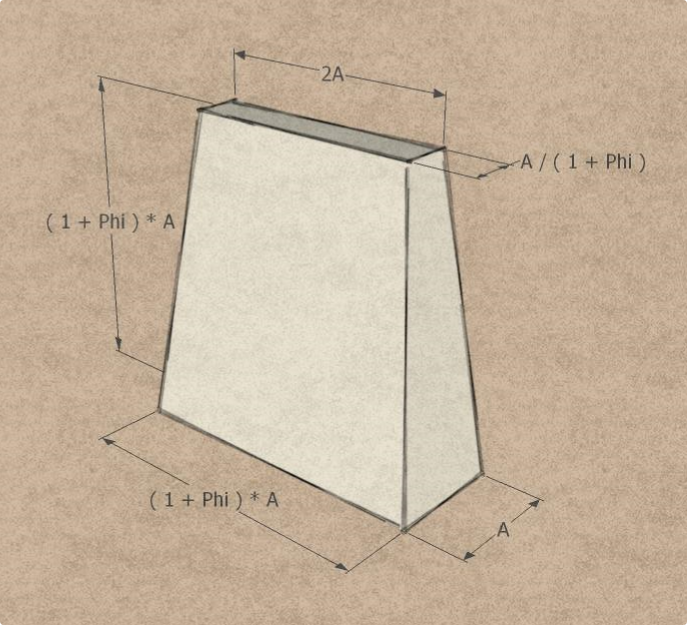In this case, the ratios of the long base to short base is (1+Phi) = Phi*Phi, rather than just Phi as before. The same ratio holds for the short base to short top. These are related to non-adjacent members of the Fibonacci sequence F(n), which can be used with a base dimension D to give the sequence of actual dimension that closely approximate the above proportions:

• Short top edge: D*F(n)
• Short base edge: D*F(n+2)
• Long base edge: D*F(n+4)

## Related Forms

This pattern is related to: